Students can Download Tamil Nadu 11th Physics Model Question Paper 1 English Medium Pdf, Tamil Nadu 11th Physics Model Question Papers helps you to revise the complete Tamilnadu State Board New Syllabus, helps students complete homework assignments and to score high marks in board exams.

## TN State Board 11th Physics Model Question Paper 1 English Medium

General Instructions:

1. The question paper comprises of four parts.
2. You are to attempt all the parts. An internal choice of questions is provided wherever applicable.
3. All questions of Part I, II, III and IV are to be attempted separately.
4. Question numbers 1 to 15 in Part I are Multiple Choice Questions of one mark each.
These are to be answered by choosing the most suitable answer from the given four alternatives and writing the option code and the corresponding answer
5. Question numbers 16 to 24 in Part II are two-mark questions. These are to be answered in about one or two sentences.
6. Question numbers 25 to 33 in Part III are three-mark questions. These are to be answered in about three to five short sentences.
7. Question numbers 34 to 38 in Part IV are five-mark questions. These are to be answered in detail Draw diagrams wherever necessary.

Time: 3 Hours
Maximum Marks: 70

PART – I

Answer all the questions: [15 × 1 = 15]

Question 1.
A cyclist moving on a circular track of radius 40 m completes half a revolution in 40 sec average velocity is ………………..
(a) 0
(b) 2 m/s
(c) 4 m/s
(d) 2π m/s
Hint:
Displacement of the cyclist in half revolution is
d = diameter of the circular track
i.e., d= 80 m
Time taken, t = 40 s
Average velocity, V == $$\frac{80}{40}$$
V = 2 m/s
(b) 2 m/sQuestion 2.
A wheel has angular acceleration of 3.0 rad/s2 and an initial angular speed of 2.00 rad/s. In a time of 2 seconds it has rotated through an angle of (in radian) ………………..
(a) 10
(b) 12
(c) 4
(d) 6
(a) 10

Question 3.
If the origin of co-ordinate system lies at the centre of mass. The sum of the moments of the masses of the system about the centre of mass is …………………
(a) May be greater than zero
(b) May be less than zero
(c) May be equal to zero
(d) Always zero
(d) Always zeroQuestion 4.
Dimensional formula for co-efficient of viscousity
(a) ML-2 T-2
(b) ML-2 T-1
(c) ML-1 T-1
(d) M-1 L-1 T-1
(c) ML-1 T-1

Question 5.
Action and reaction …………………
(a) Acts on same object
(b) Acts on two different objects
(c) Have resultant not zero
(d) Acts on the same direction
(b) Acts on two different objectsQuestion 6.
A spring is stretched by applying load to its free end. The strain produced in the spring is …………………
(a) Volumetric
(b) Shear
(c) Longitudinal
(d) Longitudinal and shear
(d) Longitudinal and shear

Question 7.
A rope is wound around a hollow cylinder of mass 3 kg and radius 40 cm. What is the angular acceleration of the cylinder if the rope is pulled with a force 30 N?
(c) 5 ms-2
(d) 25 ms-2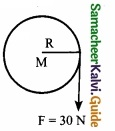Hint:
τ = F × r
Iα = F × r
MR2 × α = 30 × $$\frac{40}{100}$$; $$\frac { 3\times 40\times 40\times \alpha }{ 100\times 100 }$$ = 12
$$\frac { 3\times 16\times \alpha }{ 100 }$$ = 12; α = 25 rad/s2Question 8.
The potential energy of a simple harmonic oscillator when the particle is half way to its end point is (E is total energy) ………………
(a) $$\frac{2}{3}$$ E
(b) $$\frac{1}{3}$$ E
(c) $$\frac{1}{4}$$ E
(d) $$\frac{1}{2}$$ E
Hint:
PE = $$\frac{1}{2}$$ kx2
⇒ $$P E_{V_{2}}=\frac{1}{2} K\left(\frac{A}{2}\right)^{2}=\frac{1}{4}\left(\frac{1}{2} K A^{2}\right)$$
(c) $$\frac{1}{4}$$ E

Question 9.
A particle executes simple harmonic motion with an angular velocity and maximum acceleration of 3.5 rad/s and 7.5 m/s2 respectively. Amplitude of the oscillation is ………………
(a) 0.36
(b) 0.28
(c) 0.61
(d) 0.53
Hint:
x = A sin ωt
∴ a = $$\frac { d^{ 2 }x }{ dt^{ 2 } }$$ = -Aω2 sinωt
∴Maximum acceleration |amax| = Aω2
Now Aω2 = 7.5
A = $$\frac { 7.5 }{ \omega ^{ 2 } }$$ = $$\frac { 7.5 }{ (3.5)^{ 2 } }$$ = 0.61
(c) 0.61Question 10.
If the tension and diameter of a sonometer wire of fundamental frequency n is doubled and density is halved, then its fundamental frequency will become ………………….
(a) $$\frac{n}{4}$$
(b) $$\sqrt{2n}$$
(c) n
(d) $$\frac { n }{ \sqrt { 2 } }$$
Hint:(c) n

Question 11.
The theory of refrigerator is based on …………….
(a) Joule-Thomson effect
(b) Newton’s particle theory
(c) Joule’s effect
(d) None of the above
(d) None of the aboveQuestion 12.
Work done by 0.1 mole of a gas at 27°C to double its volume at constant pressure is ………………..
(a) 54 cal
(b) 60 cal
(c) 546 cal
(d) 600 cal
Hint:
Workdone (W) = – p.dv = nRT
= 0.1 × (0.2 cal) × (273 + 27) = 0.1 × 2 × 300
W = 60 cal
(b) 60 cal

Question 13.
When a lift is moving upwards with acceleration a, then time period of simple pendulum in it will ………………..
(a) 2π$$\sqrt { \frac { 1 }{ g+a } }$$
(b) 2π$$\sqrt { \frac { g+a }{ l } }$$
(c) $$\frac{1}{2π}$$$$\sqrt { \frac { 1 }{ g+a } }$$
(d) $$\frac{1}{2π}$$$$\sqrt { \frac { g+a }{ l } }$$
(a) 2π$$\sqrt { \frac { 1 }{ g+a } }$$

Question 14.
A disc is rotating with angular speed ω. If a child sits on it, what is conserved?
(a) Linear momentum
(b) Angular momentum
(c) Kinetic energy
(d) Potential energy
(b) Angular momentumQuestion 15.
The vectors $$\vec { A }$$ and $$\vec { B }$$ are such that |$$\vec { A }$$ + $$\vec { B }$$| = |$$\vec { A }$$ – $$\vec { B }$$|. The angle between the two vector is ………………..
(a) 45°
(b) 60°
(c) 75°
(d) 90°
Hint:
The angle between two vector is always 90°.
(d) 90°

PART – II

Answer any six questions in which Q. No 23 is compulsory. [6 × 2 = 12]

Question 16.
Velocity – time graph of a moving object is shown below. What is the acceleration of the object? Also draw displacement – time graph for the motion of the object?
The given graph shows that the velocity of the object is constant. That is, the velocity of the object is not changing, so the acceleration of the object is zero. Since the acceleration of an object is given by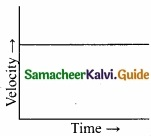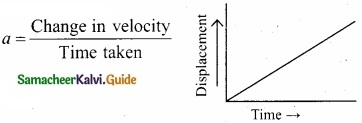Displacement – time graph for the motion of the object is shown in the figure above.

Question 17.
Can a body subjected to a uniform acceleration always move in a straight line?
It will be a straight line in one dimensional motion but not applicable for two dimensional motion because the projectile has a parabolic path but it has a uniform acceleration.Question 18.
Calculate the viscous force on a ball of radius 1mm moving through a liquid of viscosity 0.2 Nsm-2 at a speed of 0.07 ms
Radius of the ball (a) = 1mm = 1 × 10-3m
Co-effecient of viscosity of liquid (η) = 0.2 Nsm-2
Speed of the ball (v) = 0.07 ms-1
According to Stoke’s law
Viscous force F = 6 π η av
= 6 × 3.14 × 1 × 10-3 × 0.2 × 0.07
= 0.26376 × 10-3 = 2.64 × 10-4N

Question 19.
Calculate the work done by a force of 30 N in lifting a load of 2 kg to a height of 10 m (g = 10ms-2)
Given data: F = 30 N, load (m) 2 kg; height = 10m, g = 10 ms-2
Gravitational forcc F = mg = 30 N
The distance moved h = 10 m
Work done on the object W = Fh = 30 × 10 = 300 JQuestion 20.
Why are shockers used in automobiles like car?
In the event of jump or jerk, the lime of action of force increases. Since the product of force aid time is constant in a given situation. therefore the force decreases.

Question 21.
Assuming the earth to be a sphere of uniform mass density, how much would a body weigh half way down to the centre of the earth if it weighted 250 N on the surface?
As gd = g (1 – $$\frac{d}{R}$$) ⇒ mgd = mg(1 – $$\frac{d}{R}$$)
Here d = $$\frac{R}{2}$$
∴mgd = (250) × (1 – $$\frac { R/2 }{ R }$$) = 250 × $$\frac{1}{2}$$ = 125NQuestion 22.
How do you deduce that two vectors are perpendicular?
If two vectors $$\vec { A }$$ and $$\vec { B }$$ are perpendicular to each other than their scalar product $$\vec { A }$$.$$\vec { B }$$ = O because cos 90° = 0. Then the vectors $$\vec { A }$$ and $$\vec { B }$$ are said to be mutually orthogonal.

Question 23.
An air bubble of radius r in water is at a depth of h below the water surface at some instant. If P is atmospheric pressure and d and T are the density and surface tension of ater respectively. Calculate the pressure P inside the bubble?
Excess ot pressure inside the air bubble in water = $$\frac{2T}{r}$$
∴ Total pressure inside the air bubble
= atmospheric pressure + pressure due to liquid column + Excess pressure due to surface tension
= P + hρg + $$\frac{2T}{r}$$Question 24.
Define beats?
Formation of beats: When two or more waves superimpose each other with slightly different frequencies, then a sound of periodically varying amplitude at a point is observed. This phenomenon is known as beats. The number of amplitude maxima per second is called beat frequency. If we have two sources, then their difference in frequency gives the beat frequency. Number of beats per second.
n = |f1 – f2| per second.

PART – III

Answer any six questions in which Q.No. 29 is compulsory. [6 × 3 = 18]

Question 25.
Define centripetal acceleration and give any two examples?
The acceleration that is directed towards the centre of the circle along the radius and perpendicular to the velocity of the particle is known as centripetal or radial or normal acceleration.
Example:-

1. In the case of planets revolving round the Sun or the moon revolving round the earth, the centripetal force is provided by the gravitational force of attraction between them.
2. For an electron revolving round the nucleus in a circular path, the electrostatic force of attraction between the electron and the nucleus provides the necessary centripetal force.Question 26.
Write any six properties of vector product of two vectors?
(I) The vector product of any two vectors is always another vector whose direction is perpendicular to the plane containing these two vectors, i.e., orthogonal to both the vectors $$\vec { A }$$ and $$\vec { B }$$, even though the vectors $$\vec { A }$$ and $$\vec { B }$$ may or may not be mutually orthogonal.

(II) The vector product of two vectors is not commutative, i.e., $$\vec { A }$$ × $$\vec { B }$$ ≠ $$\vec { B }$$ × $$\vec { A }$$. But, $$\vec { A }$$ × $$\vec { B }$$ = – $$\vec { B }$$ × $$\vec { A }$$ .
Here it is worthwhile to note that |$$\vec { A }$$ × $$\vec { B }$$| = |$$\vec { B }$$ × $$\vec { A }$$| = AB sin θ i.e., in the case of the product vectors $$\vec { A }$$ × $$\vec { B }$$ and $$\vec { B }$$ × $$\vec { A }$$, the magnitudes are equal but directions are opposite to each other.

(III) The vector product of two vectors will have maximum magnitude when sin θ = 1, i.e., θ = 90° i.e., when the vectors $$\vec { A }$$ and $$\vec { B }$$ are orthogonal to each other.
($$\vec { A }$$ × $$\vec { B }$$)max = AB$$\hat { n }$$

(IV) The vector product of two non-zero vectors will be minimum when sin θ = 0, i.e., θ = 0° or θ = 180°
($$\vec { A }$$ × $$\vec { B }$$)min = 0
i.e., the vector product of two non-zero vectors vanishes, if the vectors are either parallel or antiparallel.

(V) The self-cross product, i.e., product of a vector with itself is the null vector
$$\vec { A }$$ × $$\vec { A }$$ = AA sin 0° $$\hat { n }$$ = $$\vec { 0 }$$
In physics the null vector $$\vec { 0 }$$ is simply denoted as zero.

(VI) The self-vector products of unit vectors are thus zero.
$$\hat { i }$$ × $$\hat { i }$$ = $$\hat { j }$$ × $$\hat { j }$$ = $$\hat { k }$$ × $$\hat { k }$$ = 0Question 27.
Show that the pressure of the gas is equal to two third of mean kinetic energy per unit volume?
The internal energy of the gas is given by
U = $$\frac{3}{2}$$ NkT
The above equation can also be written as
U = $$\frac{3}{2}$$ PV
Since PV = NkT
P = $$\frac{2}{3}$$ $$\frac{U}{V}$$ = $$\frac{2}{3}$$ u
From the equation (1), we can state that the pressure of the gas is equal to two thirds of internal energy per unit volume or internal energy density (u = $$\frac{U}{V}$$)
Writing pressure in terms of mean kinetic energy density using equation.
P = $$\frac{1}{3}$$ nm$$\overline { V^{ 2 } }$$ = $$\frac{1}{3}$$ ρ$$\overline { V^{ 2 } }$$
where ρ = nm = mass density (Note n is number density)
Multiply and divide R.H.S of equation (2) by 2, we get
P = $$\frac{2}{3}$$($$\frac{ρ}{2}$$ $$\overline { V^{ 2 } }$$)
P = $$\frac{2}{3}$$ $$\overline { KE }$$
From the equation (3), pressure is equal to $$\frac{2}{3}$$ of mean kinetic energy per unit volume.Question 28.
Derive an expression for gravitational potential energy?
The gravitational tbrce is a conservative force and hence we can define a gravitational potential energy associated with this conservative force field.
Two masses m1 and m2, are initially separated by a distance r’. Assuming m1 to be fixed in its position. work must be done on m2 to move the distance from r’ to r.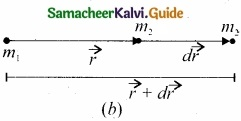To move the mass m2, through an infinitesimal displacement d$$\vec { r }$$ from $$\vec { r }$$ to $$\vec { r }$$ + d$$\vec { r }$$ , work has to be done externally. This infinitesimal work is given by
dW = $$\vec { F }$$ext . d$$\vec { r }$$ ……………….. (1)
The work is done against the gravitational force, therefore,
$$\vec { F }$$ext = $$\frac { Gm_{ 1 }m_{ 2 } }{ r^{ 2 } } \hat { r }$$ ………………. (2)
Substituting equation (2) in (1), we get
dW = $$\frac { Gm_{ 1 }m_{ 2 } }{ r^{ 2 } } \hat { r }$$.d$$\vec { r }$$ ………………… (3)
d$$\vec { r }$$ = dr $$\hat { r }$$ ⇒ dW = $$\frac { Gm_{ 1 }m_{ 2 } }{ r^{ 2 } } \hat { r }$$.(dr $$\hat { r }$$)
$$\hat { r }$$.$$\hat { r }$$ = 1 (Since both are unit vectors)
∴ dW = $$\frac { Gm_{ 1 }m_{ 2 } }{ r^{ 2 } }$$ dr …………….. (4)
Thus the total work done for displacing the particle from r’ to r isThis work done W gives the gravitational potential energy difference of the system of masses and m1 and m2 when the separation between them are r and r’ respectively.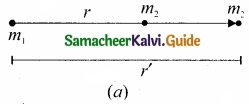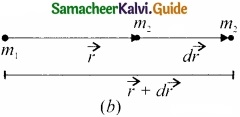Question 29.
A satellite orbiting the Earth in a circular orbit of radius 1600 km above the surface of the Earth. What is the acceleration experienced by satellite due to Earth’s gravitational force?
g’ = g(1 – $$\frac { 2h }{ R_{ e } }$$)
= g($$\frac { 1-2\times 1600\times 10^{ 3 } }{ 6400\times 10^{ 3 } }$$) = g(1 – $$\frac{2}{4}$$)
g’ = g (1 – $$\frac{1}{2}$$) = $$\frac{g}{2}$$
g’ = g (1- $$\frac{1}{2}$$) = $$\frac{g}{2}$$
g’ = $$\frac{8}{2}$$ (or) g’ = $$\frac{9.8}{2}$$ = 4.9ms-2Question 30.
Explain the v ariation of a g with latitude?
When an object is on the surface fo the Earth, it experiences a centrifugal force that depends on the latitude of the object on Earth. If the Earth were not spinning, the force on the object would have been mg. However, the object experiences an additional centrifugal force due to spinning of the Earth.
This centrifugal force is given by mωR’.
$$\mathrm{OP}_{z}, \cos \lambda=\frac{\mathrm{PZ}}{\mathrm{OP}}=\frac{\mathrm{R}^{\prime}}{\mathrm{R}}$$
R’ = R cos λ
where λ is the latitude. The component of centrifugal acceleration experienced by the object in the direction opposite to g is
$$a_{\mathrm{PQ}}=\omega^{2} \mathrm{R} \cos \lambda=\omega^{2} \mathrm{R} \cos ^{2} \lambda$$
Since R’ = R cos λ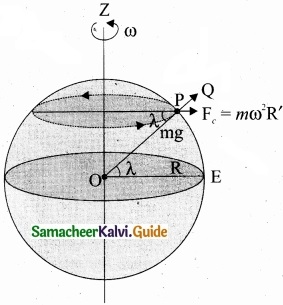Therefore, g = g – ω2R cos2 λ
From the above expression, we can infer that at equator, λ = 0, g’ = g – ω2R. The acceleration due to gravity is minimum. At poles λ = 90; g’ = g, it is maximum. At the equator, g’ is minimum.Question 31.
A bullet of mass 50g is fired from below into a suspended object of mass 450 g. The object rises through a height of 1.8 m with bullet remaining inside the object. Find the speed of the bullet. Take g = 10 ms-2
m1 = 50 g = 0.05 kg; m2 = 450 g = 0.45 kg
The speed of the bullet is u1 The second body is at rest (u2 = 0). Let the common velocity of the bullet and the object after the bullet is embedded into the object is v.
v = $$\frac{m_{1} u_{1}+m_{2} u_{2}}{\left(m_{1}+m_{2}\right)}$$
v = $$\frac{0.05 u_{1}+(0.45 \times 0)}{(0.05+0.45)}$$ = $$\frac{0.05}{0.50}$$u1
The combined velocity is the initial velocity for the vertical upward motion of the combined bullet and the object. From second equation of motion,
v = $$\sqrt{2gh}$$
v = $$\sqrt{2 \times 10 \times 1.8}$$ = $$\sqrt{36}$$
v = 6 ms-1
Substituting this in the above equation, the value of u1 is
6 = $$\frac{0.05}{0.50}$$u1 or u1 = $$\frac{0.05}{0.50}$$ × 6 = 10 × 6
u1 = 60ms-1Question 32.
If the piston of a container is pushed fast inward. Will the ideal gas equation be valid in the intermediate stage? If not, why?
When the piston is compressed so quickly that there is no time to exchange heat to the surrounding, the temperature of the gas increases rapidly. In this intermediate stage the ideal gas equation be not valid. Because this equation can be relates the pressure, volume and temperature of thermodynamic system at equilibrium.Question 33.
Calculate how many times more intense is 90 dB sound compared to 40 dB sound?
Given Data:
L = log $$\frac { I }{ I_{ 0 } }$$ = log I – log I0
We get 90 dB = 9 B = log I1 – log I0 ……………….. (1)
40 dB = 4 B = logI2 – logI0 ………………….. (2)
Subtract (2) from (1)
50 dB = 5B = log I1 – logI2
5 = log10 ($$\frac{I_{1}}{I_{2}}$$)
$$\frac{I_{1}}{I_{2}}$$ = 105

PART – IV

Answer all the questions. [ 5 × 5 = 25]

Question 34 (a)
Obtain an expression for the time period T of a simple pendulum. The time period T depends on

1. Mass of the bob(m)
2. Length of the pendulum (l)
3. Acceleration due to gravity (g) at the place where the pendulum is suspended, (constant k = 2π)

Example:
An expression for the time period T of a simple pendulum can be obtained by using this method as follows.
Let true period T depend upon

1. Mass m of the bob
2. Length l of the pendulum and
3. Acceleration due to gravity g at the place where the pendulum is suspended. Let the constant involved is k = 2π.

Solution:Here k is the dimensionless constant. Rewriting the above equation with dimensions.Comparing the powers of M, L and T on both sides, a = 0, b + c = 0, -2c = 1
Solving for a, b and c ⇒ a = 0, b = 1/2, and c = -1/2
From the above equation
T = $$\mathrm{k} \mathrm{m}^{0} l^{1}=g^{-1}-2$$
T = $$k\left(\frac{1}{g}\right)^{1}$$ = $$k \sqrt{1 / g}$$
Experimentally k = 2π, hence
T = $$2 \pi \sqrt{1 / g}$$

[OR]

(b) Obtain an expression for the escape speed in detail?
Consider an object of mass M on the surface of the Earth. When it is thrown up with an initial speed vi the initial total energy of the object is
$$\mathrm{E}_{i}=\frac{1}{2} \mathrm{M} v_{i}^{2}-\frac{\mathrm{GMM}_{\mathrm{E}}}{\mathrm{R}_{\mathrm{E}}}$$ ………………. (1)
where, ME is the mass of the Earth and RE the radius of the Earth.
The term $$-\frac{\mathrm{GMM}_{\mathrm{E}}}{\mathrm{R}_{\mathrm{E}}}$$ is the potential energy of the mass M.
When the object reaches a height far away from Earth and hence treated as approaching infinity, the gravitational potential energy becomes zero [U(∞) = 0] and the kinetic energy becomes zero as well. Therefore the final total energy of the object becomes zero. This is for minimum energy and for minimum speed to escape. Otherwise kinetic energy can be nonzero.
EF = 0
Ei = Ef ……………….. (2)
Substituting (1) in (2) we get,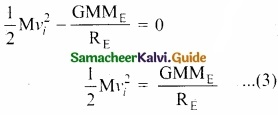Consider the escape speed, the minimum speed required by an object to escape Earth’s gravitational field, hence replace vi with ve i.e..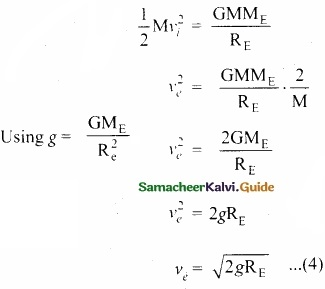From equation (4) the escape speed depends on two factors acceleration due to gravity and radius of the Earth. It is completely independent of the mass of the object. By substituting the values of g (9.8 ms-2) and Re = 6400 km, the escape speed of the Earth is ve = 11.2 kms-1. The escape speed is independent of the direction in which the object is thrown. Irrespective of whether the object is thrown vertically up, radially outwards or tangentially it requires the same initial speed to escape Earth’s gravity.Question 35 (a).
Derive an expression for loss of kinetic energy in perfect inelastic collision?
In perfectly inelastic collision, the loss in kinetic energy during collision is transformed to another form of energy like sound, thermal, heat, light etc. Let KEi be the total kinetic energy before collision and KEf be the total kinetic energy after collision.
Total kinetic energy before collision,
$$\mathrm{KE}_{i}=\frac{1}{2} m_{1} u_{1}^{2}+\frac{1}{2} m_{2} u_{2}^{2}$$ …………….. (1)
Total kinetic energy after collision
KEf = $$\frac{1}{2}$$ (m1 + m2)v2 …………….. (2)
Then the loss of kinetic energy is
Loss of KE, ∆Q = KEf – KEi = $$\frac{1}{2}$$ (m1 + m2)v2 – $$\frac{1}{2}$$ m1 u12 – $$\frac{1}{2}$$ m2 u22 ………………. (3)
Substituting equation v = $$\frac{m_{1} u_{1}+m_{2} u_{2}}{\left(m_{1}+m_{2}\right)}$$ in equation (3), and on simplying (expand v by using the algebra) (a + b)2 = a2 + b2 + 2ab, we get
Loss of KE, ∆Q = $$\frac{1}{2}$$ $$\left(\frac{m_{1} m_{2}}{m_{1}+m_{2}}\right)$$ (u1 – u2)2

(b) A shell of mass 200 gm is ejected from a gun of mass 4 kg by an explosion that generates 1.05 kJ of energy. Calculate the initial velocity of the shell?
Given Data :
m = 200 gm = 0.2 kg; M = 4 kg.
Energy generated = 1.05 KJ = 1.05 × 103 J
According to law of Conservation of linear momentum
mv = Mv’
∴v’ = ($$\frac{m}{M}$$) v
Total K.E of the gun and bullet[OR]

(c) State parallel axis theorem?
Parallel axis theorem: Parallel axis theorem states that the moment of inertia of a body about any axis is equal to the sum of its moment of inertia about a parallel axis through its center of mass and the product of the mass of the body and the square of the perpendicular distance between the two axes.

If IC is the moment of inertia of the body of mass M about an axis passing through the center of mass, then the moment of inertia I about a parallel axis at a distance d from it is given by the relation,
I = IC + Md2

(d) Calculate the moment of inertia of uniform circular disc of mass 500 G radius 10 cm about

1. The diameter of the disc
2. The axis, tangent to the disc and parallel to its diameter
3. The axis through the centre of the disc and perpendicular to its plane

1. Given Data: M = 500 g = 0.5 kg. R = 10 cm = 10 × 10-2 m
Moment of inertia of disc about diameter = Id = $$\frac{1}{4}$$ MR2
Id = $$\frac{1}{4}$$ × 0.5 × 0.1 kg m2 = 0.0125 kg m2

2. Apply a parallel axes theorem, moment of inertia of the disc about a tangent to the disc and parallel to the diameter of the disc
= $$\frac{1}{4}$$ MR2 + MR2 = $$\frac{5}{4}$$ MR2 = $$\frac{5}{4}$$ × 0.5 × 1
= 0.0625 kgm2

3. Moment of inertia of the disc about an axis passing through the centre of disc and perpendicular to the plane of the disc
= $$\frac{1}{2}$$ MR2 = $$\frac{1}{2}$$ × 0.5 × 0.1 = 0.025 kgm2Question 36 (a).
Prove the law of conservation of linear momentum. Use it to find the recoil velocity of a gun when a bullet is fired from it?
In nature, conservation laws play a very important role. The dynamics of motion of bodies can be analysed very effectively using conservation laws. There are three conservation laws in mechanics. Conservation of total energy, conservation of total linear momentum, and conservation of angular momentum. By combining Newton’s second and third laws, we can derive the law of conservation of total linear momentum.

When two particles interact with each other, they exert equal and opposite forces on each other. The particle 1 exerts force $$\vec { F }$$12 on particle 2 and particle 2 exerts an exactly equal and opposite force $$\vec { F }$$12 on particle 1 according to Newton’s third law.
$$\vec { F }$$21 = –$$\vec { F }$$12 …………… (1)
In terms of momentum of particles, the force on each particle (Newton’s second law) can be written as
$$\vec { F }$$12 = $$\frac{d \bar{p}_{1}}{d t}$$ and $$\vec { F }$$21 = $$\frac{d \vec{p}_{2}}{d t}$$ ……………… (2)

Here $$\vec { P }$$1 is the momentum of particle 1 which changes due to the force $$\vec { F }$$12 exerted by particle 2. Further $$\vec { P }$$2 is the momentum of particle 2. This changes due to $$\vec { F }$$21 exerted by particle 1.
Substitute equation (2) in equation (1)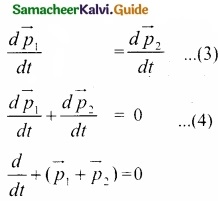It implies that $$\vec { P }$$1 + $$\vec { P }$$2 = (constant vector always)

$$\vec { P }$$1 + $$\vec { P }$$2 is the total linear momentum of the two particles ( $$\vec { P }$$tot = $$\vec { P }$$1 + $$\vec { P }$$2). It is also called as total linear momentum of the system. Flere, the two particles constitute the system. From this result, the law of conservation of linear momentum can be stated as follows.

If there are no external forces acting on the system, then the total linear momentum of the system ( $$\vec { P }$$tot) is always a constant vector. In other words, the total linear momentum of the system is conserved in time. Here the word ‘conserve’ means that $$\vec { P }$$1 and $$\vec { P }$$2 can vary,
in such a way that $$\vec { P }$$1 + $$\vec { P }$$2 is a constant vector.

The forces $$\vec { F }$$12 and $$\vec { F }$$12 are called the internal forces of the system, because they act only between the two particles. There is no external force acting on the two particles from outside. In such a case the total linear momentum of the system is a constant vector or is conserved.

To find the recoil velocity of a gun when a bullet is fired from it:
Consider the firing of a gun. Here the system is Gun + bullet. Initially the gun and bullet are at rest, hence the total linear momentum of the system is zero. Let $$\vec { P }$$1 be the momentum of the bullet and $$\vec { P }$$2 the momentum of the gun before firing. Since initially both are at rest.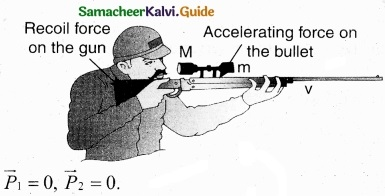$$\vec { P }$$1 = 0, $$\vec { P }$$2 = 0.

Total momentum before firing the gun is zero, $$\vec { P }$$1 + $$\vec { P }$$2 = 0

According to the law of conservation of linear momentum, total linear momemtum has to be zero after the firing also.

When the gun is fired, a force is exerted by the gun on the bullet in forward direction. Now the momentum of the bullet changes from $$\vec { P }$$1 + $$\vec { P }$$2. To conserve the total linear momentum of the system, the momentum of the gun must also change from $$\vec { P }$$2 to $$\vec { P }$$2. Due to the conservation of linear momentum, $$\vec { P }$$1+ $$\vec { P }$$2‘= 0. It implies that $$\vec { P }$$1‘ = –$$\vec { P }$$2 the momentum of the gun is exactly equal, but in the opposite direction to the momentum of the bullet. This is the reason after firing, the gun suddenly moves backward with the momentum $$\vec { P }$$2. It is called ‘recoil momentum’. This is an example of conservation of total linear momentum.

[OR]

(b) Derive an expression for escape speed?
Consider an object of mass M on the surface of the Earth. When it is thrown up with an initial speed v;, the initial total energy of the object is
$$E_{i}=\frac{1}{2} M v_{i}^{2}-\frac{G M M_{E}}{R_{E}}$$ ………………. (1)
where, ME is the mass of the Earth and RE the radius of the Earth. The term $$-\frac{\mathrm{GMM}_{\mathrm{E}}}{\mathrm{R}_{\mathrm{E}}}$$ is the potential energy of the mass M.

When the object reaches a height far away from Earth and hence treated as approaching infinity, the gravitational potential energy becomes zero [U(∞) = 0] and the kinetic energy becomes zero as well. Therefore the final total energy of the object becomes zero. This is for minimum energy and for minimum speed to escape. Otherwise kinetic energy can be nonzero.
Ef = 0

According to the law of energy conservation,
Ei – Ef = 0 …………….. (2)

Substituting (1) in (2) we get,
$$\frac{1}{2} \mathrm{M} v_{i}^{2}-\frac{\mathrm{GMM}_{\mathrm{E}}}{\mathrm{R}_{\mathrm{F}}}=0$$
$$\frac{1}{2} \mathrm{M} v_{i}^{2}=\frac{\mathrm{GMM}_{\mathrm{E}}}{\mathrm{R}_{\mathrm{E}}}$$ …………….. (3)

Consider the escape speed, the minimum speed required by an object to escape Earth’s gravitational field, hence replace vi with ve, i.e.,From equation (4) the escape speed depends on two factors acceleration due to gravity and radius of the Earth. It is completely independent of the mass of the object. By substituting the values of g (9.8 ms-2) and Re = 6400 km, the escape speed of the Earth is ve = 11.2 kms-1. The escape speed is independent of the direction in which the object is thrown. Irrespective of whether the object is thrown vertically up, radially outwards or tangentially it requires the same initial speed to escape Earth’s gravity.Question 37 (a).
Explain in detail Newton’s law of cooling?
Newton’s law of cooling: Newton’s law of cooling states that the rate of loss of heat of a body is directly proportional to the difference in the temperature between that body and its surroundings.
$$\frac{dQ}{dt}$$ ∝ (T – Ts) …………….. (1)

The negative sign indicates that the quantity of heat lost by liquid goes on decreasing with time. Where,

T = Temperature of the object
Ts = Temperature of the surrounding

From the graph in figure it is clear that the rate of cooling is high initially and decreases with falling temperature.

Let us consider an object of mass m and specific heat capacity s at temperature T. Let Ts be the temperature of the surroundings. If the temperature falls by a small amount of T in time dt, then the amount of heat lost is,
dQ = msdT ………………. (2)
Dividing both sides of equation (2) by dt
$$\frac{dQ}{dt}$$ = $$\frac{msdT}{dt}$$ ……………….. (3)
From Newton’s of cooling
$$\frac{dQ}{dt}$$ ∝ (T – Ts)
$$\frac{dQ}{dt}$$ = -a(T – Ts) ……………….. (4)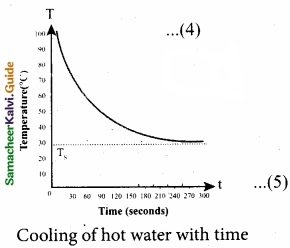Where a is some positive constant.
From equation (3) and (4)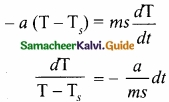Integrating equation (5) on both sides,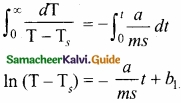Where b1 is the constant of integration. Taking exponential both sides, we get
$$\mathrm{T}=\mathrm{T}_{\mathrm{s}}+b_{2} e^{\frac{-a}{m s}}$$ ……………. (6)
Here b2 = eb1 = Constant

[OR]

(b) Derive an expression for pressure exerted by the gas on the wall of the container?
Expression for pressure exerted by a gas:
Consider a monoatomic gas of N molecules each having a mass m inside a cubical container of side l.
The molecules of the gas are in random motion. They collide with each other and also with the walls of the container. As the collisions are elastic in nature, there is no loss of energy, but a change in momentum occurs.The molecules of the gas exert pressure on the walls of the container due to collision on it. During each collision. the molecules impart certain momentum to the wall. Due to transfer of momentum, the walls experience a continuous force. The force experienced per unit area of the walls of the container determines the pressure exerted by the gas. It is essential to determine the total momentum transferred by the molecules in a short interval of time.

A molecule of mass in moving with a velocity $$\vec { v }$$ having components (vx vy, vz) hits the right side wall. Since we have assumed that the collision is elastic, the particle rebounds with sanie speed and its x-component is reversed. This is shown in the figure. The components of velocity of the molecule after collision are (-vx, vy, vz)
The x-component of momentum of the molecule bêfore collision = mvx
The x-component of momentum of the molecule after collision = -mvx
The change in momentum of the molecule in x direction
= Final momentum – initial momentum = -mvx – mvx = -2mvx
According to law of conservation of linear momentum, the change in momentum of the wall = 2mvx

The number of molecules hitting the right side wall in a small interval of time ∆t.

The molecules within the distance of vx ∆t from the right side wall and moving towards the right will hit the wall in the time interval &. The number of molecules that will hit the right side wall in a time interval ∆t is equal to the product of volume (Avx∆t) and number density of the molecules n). Here A is area of the wall and ii is number of molecules per
unit volume $$\frac{N}{V}$$ We have assumed that the number density is the same throughout the cube.

Not all the n molecules will move to the right, therefore on an average only half of the n molecules move to the right and the other half moves towards left side.

Te no.of molecules that hit the right side wall in a time interval ∆t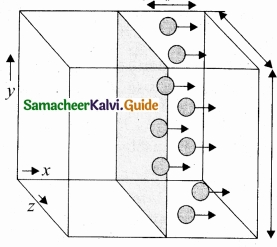= $$\frac{n}{2} \mathrm{A} v_{x} \Delta t$$ ……………….. (1)
In the same interval of time ∆t, the total momentum transferred by the molecules
$$\Delta \mathrm{P}=\frac{n}{2} \mathrm{A} v_{x} \Delta t \times 2 m v_{x}=\mathrm{A} v_{x}^{2} m n \Delta t$$ ……………….. (2)

From Newton’s second law, the change in momentum in a small interval of time gives rise to force.
The force exerted by the molecules on the wall (in magnitude)

F = $$\frac{\Delta p}{\Delta t}=n m \mathrm{A} v_{x}^{2}$$ ……………….. (3)
Pressure P = force divided by the area of the wall

P = $$\frac{F}{A}$$ = nmv2x …………………. (4)
Since all the molecules are moving completely in random manner, they do not have same speed. So we can replace the term v2x by the average $$\bar{v}_{x}^{2}$$ in equation (4)

P = nm$$\bar{v}_{x}^{2}$$ ………………… (5)

Since the gas is assumed to move in random direction, it has no preferred direction of motion (the effect of gravity on the molecules is neglected). It implies that the molecule has same average speed in all the three direction. So, $$\bar{v}_{x}^{2}$$ = $$\bar{v}_{y}^{2}$$ = $$\bar{v}_{z}^{2}$$. The mean square speed is written as

$$\bar{v}^{2}$$ = $$\bar{v}_{x}^{2}$$ + $$\bar{v}_{y}^{2}$$ + $$\bar{v}_{z}^{2}$$ = 3$$\bar{v}_{x}^{2}$$
$$\bar{v}_{x}^{2}$$ = $$\frac{1}{3}$$ $$\bar{v}^{2}$$
Using this in equation (5), we get
P = $$\frac{1}{3}$$nm $$\bar{v}^{2}$$ or P = $$\frac{1}{3}$$ $$\frac{N}{V}$$ m$$\bar{v}^{2}$$ as [n = $$\frac{N}{V}$$] ……………… (6)Question 38 (a).
Explain with graphs the difference between work done by a constant force and by a variable force. Arrive at an expression for power and velocity. Give some examples for the same?
Work done by a constant force: When a constant force F acts on a body, the small work done (dW) by the force in producing a small displacement dr is given by the relation,
dW = (F cos θ) dr ………………. (1)
The total Work done in producing a displacement from initial position ri to final position rf is,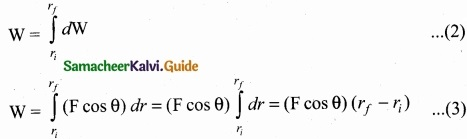The graphical representation of the work done by a constant force is shown in figure given below. The area under the graph shows the work done by the constant force.

Work done by a variable force:
When the component of a variable force F acts on a body, the small work done (dW) by the force in producing a small displacement dr is given by the relation.dW = F cos θ dr [F cos θ is the component of the variable force F]
where, F and θ are variables. The total work done for a displacement from initial position ri to final position rf is given by the relation,
W = $$\int_{r_{i}}^{r_{f}} d \mathrm{W}=\int_{r_{i}}^{r_{f}} \mathrm{F} \cos \theta d r$$ ………………. (4)

A graphical representation of the work done by a variable force is shown in figure given below. The area under the graph is the work done by the variable force.

Expression for power and velocity

The work done by a force $$\vec { F }$$ for a displacement $$\bar { dr }$$ is
W = ∫$$\vec { F }$$.$$\vec { dr }$$ ……………. (1)
Left hand side of the equation (1) can be written as
W = ∫dW = ∫$$\frac{dW}{dt}$$ (multiplied and divided by dt) ………………… (2)
Since, velocity is $$\vec { v }$$ = $$\frac{d \vec{r}}{d t}$$; $$\vec { dr }$$ = $$\vec { v }$$ dt. Right hand side of the equation (I) can be written as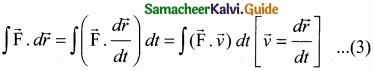Substituting equation (2) and equation (3) in equation (1), we getThis relation is true for any arbitrary value of di. This implies that the term within the bracket must be equal to zero, i.e.,
$$\frac{dW}{dt}$$ – $$\vec { F }$$.$$\vec { v }$$ = 0 Or $$\frac{dW}{dt}$$ = $$\vec { F }$$.$$\vec { v }$$
Hence power P = $$\vec { F }$$.$$\vec { v }$$

[OR]

(b) Explain in detail Newton’s law of cooling?
Newton’s law of cooling: Newton’s law of cooling states that the rate of loss of heat of a body is directly proportional to the difference in the temperature between that body and its surroundings.
$$\frac{dQ}{dt}$$ ∝ (T – Ts)
The negative sign indicates that the quantity of heat lost by liquid goes on decreasing with time. Where,
T = Temperature of the object
Ts = Temperature of the surrounding
From the graph in figure it is clear that the rate of cooling is high initially and decreases with falling temperature.
Let us consider an object of mass m and specific heat capacity s at temperature T. Let Ts be the temperature of the surroundings. If the temperature falls by a small amount dT in
time dt, then the amount of heat lost is,
dQ = msdT ………………. (2)
Dividing both sides of equation (2) by dt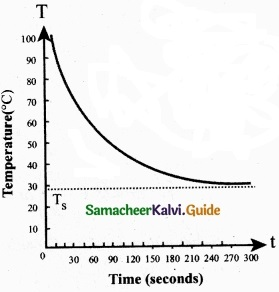$$\frac{dQ}{dt}$$ = $$\frac{msdT}{dt}$$ ……………….. (3)
From Newton’s law of cooling
$$\frac{dQ}{dt}$$ ∝ (T – Ts)
$$\frac{dQ}{dt}$$ -a(T – Ts) …………………. (4)
Where a is a positive constant.
From equation (3) and (4)
– a(T – Ts) = ms $$\frac{dT}{dt}$$
$$\frac{d T}{T-T_{s}}$$ = -a$$\frac{a}{ms}$$ dt ………………. (5)
Integrating equation (5) on both sides,Where b1 is the constant of integration. Taking exponential both sides we get,
$$\mathrm{T}=\mathrm{T}_{s}+b_{2} e^{\frac{-a}{m s}}$$ ………………… (6)
Here b2 = eb1 = constant